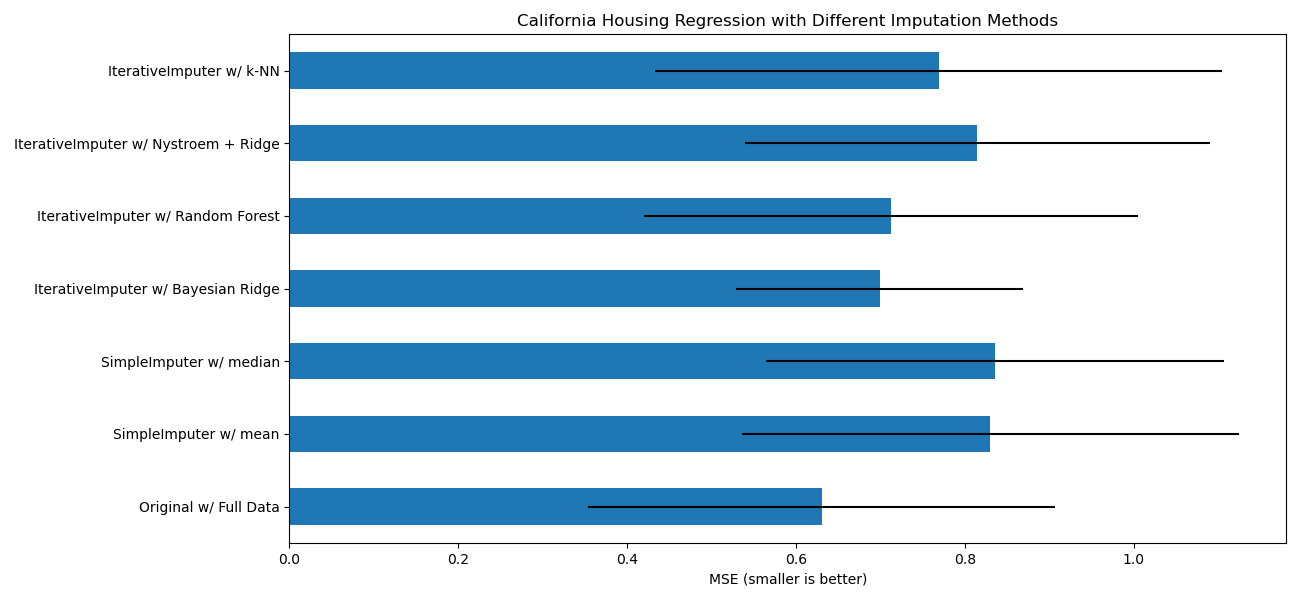# Imputing missing values with variants of IterativeImputer¶

The IterativeImputer class is very flexible - it can be used with a variety of estimators to do round-robin regression, treating every variable as an output in turn.

In this example we compare some estimators for the purpose of missing feature imputation with IterativeImputer:

Of particular interest is the ability of IterativeImputer to mimic the behavior of missForest, a popular imputation package for R.

Note that KNeighborsRegressor is different from KNN imputation, which learns from samples with missing values by using a distance metric that accounts for missing values, rather than imputing them.

The goal is to compare different estimators to see which one is best for the IterativeImputer when using a BayesianRidge estimator on the California housing dataset with a single value randomly removed from each row.

For this particular pattern of missing values we see that BayesianRidge and RandomForestRegressor give the best results.

It should be noted that some estimators such as HistGradientBoostingRegressor can natively deal with missing features and are often recommended over building pipelines with complex and costly missing values imputation strategies.import numpy as np
import matplotlib.pyplot as plt
import pandas as pd

# To use this experimental feature, we need to explicitly ask for it:
from sklearn.experimental import enable_iterative_imputer  # noqa
from sklearn.datasets import fetch_california_housing
from sklearn.impute import SimpleImputer
from sklearn.impute import IterativeImputer
from sklearn.linear_model import BayesianRidge, Ridge
from sklearn.kernel_approximation import Nystroem
from sklearn.ensemble import RandomForestRegressor
from sklearn.neighbors import KNeighborsRegressor
from sklearn.pipeline import make_pipeline
from sklearn.model_selection import cross_val_score

N_SPLITS = 5

rng = np.random.RandomState(0)

X_full, y_full = fetch_california_housing(return_X_y=True)
# ~2k samples is enough for the purpose of the example.
# Remove the following two lines for a slower run with different error bars.
X_full = X_full[::10]
y_full = y_full[::10]
n_samples, n_features = X_full.shape

# Estimate the score on the entire dataset, with no missing values
br_estimator = BayesianRidge()
score_full_data = pd.DataFrame(
cross_val_score(
br_estimator, X_full, y_full, scoring="neg_mean_squared_error", cv=N_SPLITS
),
columns=["Full Data"],
)

# Add a single missing value to each row
X_missing = X_full.copy()
y_missing = y_full
missing_samples = np.arange(n_samples)
missing_features = rng.choice(n_features, n_samples, replace=True)
X_missing[missing_samples, missing_features] = np.nan

# Estimate the score after imputation (mean and median strategies)
score_simple_imputer = pd.DataFrame()
for strategy in ("mean", "median"):
estimator = make_pipeline(
SimpleImputer(missing_values=np.nan, strategy=strategy), br_estimator
)
score_simple_imputer[strategy] = cross_val_score(
estimator, X_missing, y_missing, scoring="neg_mean_squared_error", cv=N_SPLITS
)

# Estimate the score after iterative imputation of the missing values
# with different estimators
estimators = [
BayesianRidge(),
RandomForestRegressor(
# We tuned the hyperparameters of the RandomForestRegressor to get a good
# enough predictive performance for a restricted execution time.
n_estimators=4,
max_depth=10,
bootstrap=True,
max_samples=0.5,
n_jobs=2,
random_state=0,
),
make_pipeline(
Nystroem(kernel="polynomial", degree=2, random_state=0), Ridge(alpha=1e3)
),
KNeighborsRegressor(n_neighbors=15),
]
score_iterative_imputer = pd.DataFrame()
# iterative imputer is sensible to the tolerance and
# dependent on the estimator used internally.
# we tuned the tolerance to keep this example run with limited computational
# resources while not changing the results too much compared to keeping the
# stricter default value for the tolerance parameter.
tolerances = (1e-3, 1e-1, 1e-1, 1e-2)
for impute_estimator, tol in zip(estimators, tolerances):
estimator = make_pipeline(
IterativeImputer(
random_state=0, estimator=impute_estimator, max_iter=25, tol=tol
),
br_estimator,
)
score_iterative_imputer[impute_estimator.__class__.__name__] = cross_val_score(
estimator, X_missing, y_missing, scoring="neg_mean_squared_error", cv=N_SPLITS
)

scores = pd.concat(
[score_full_data, score_simple_imputer, score_iterative_imputer],
keys=["Original", "SimpleImputer", "IterativeImputer"],
axis=1,
)

# plot california housing results
fig, ax = plt.subplots(figsize=(13, 6))
means = -scores.mean()
errors = scores.std()
means.plot.barh(xerr=errors, ax=ax)
ax.set_title("California Housing Regression with Different Imputation Methods")
ax.set_xlabel("MSE (smaller is better)")
ax.set_yticks(np.arange(means.shape))
ax.set_yticklabels([" w/ ".join(label) for label in means.index.tolist()])